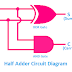Half Adder and Full adder are common examples of combinational digital logic circuits. The main characteristics of the combinational circuits, the output of the circuit depends upon the input and there are working with a loop control system. In this article, we are going to see Half Adder Circuit Diagram, Full Adder Circuit Diagram, Half Adder Truth Table, and Full Adder Truth Table.

In digital electronics, adder circuits are those used to add binary numbers. You can see the applications of adder circuits in digital calculators, computers, mobiles, etc. Also, they are used for calculating addresses. The main difference between the half adder and full adder is, half adder has two inputs and two outputs whereas the full adder has three inputs and two outputs.

Here, you can see the circuit diagram of half adder.

You can see the circuit consists of an XOR gate and a AND Gate. A and B are the inputs of the circuit. 'S' is for sum and 'C' is for Carry.

Here, you can see the truth table of Half Adder.

Half adder allows two inputs(A, B) and generates a sum and a carry. So there are four possible combinations of the input numbers.

Here, you can see the circuit diagram of full adder.

This circuit consists of two XOR gates, two AND Gates, and an OR gate. A, B, Cin are inputs for the circuit and S is for Sum, C is for Carry.

Here, you can see the truth table of full adder.

Full Adder allows three inputs(A, B, Cin) and generates a sum and a carry, so there are eight possible combinations for the input numbers.

Thank you for visiting the website. keep visiting for more updates.

Half Adder and Full Adder Circuit Diagram, Truth TableReviewed by Author on October 13, 2021 Rating: 5